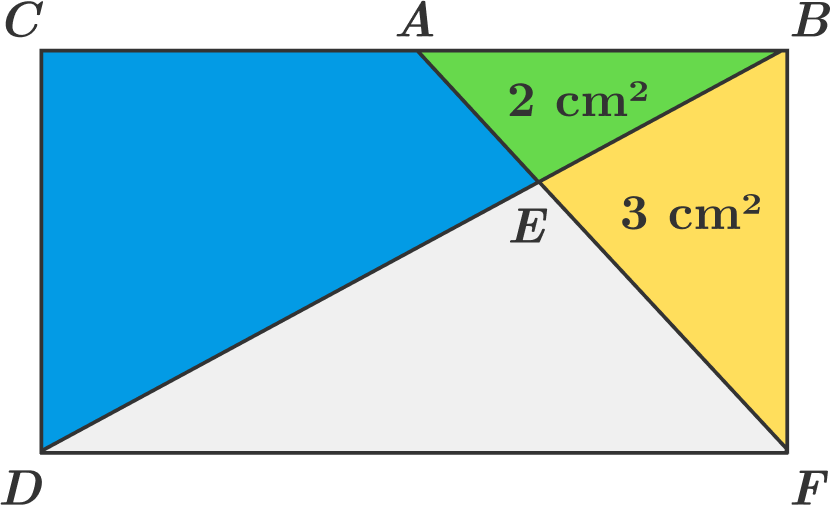# Rectangle Divisions

Geometry Level 3$BCDF$ is a rectangle. Triangle $ABE$ has an area of $2 \text{ cm}^2$. Triangle $BEF$ has an area of $3 \text{ cm}^2$.

Find the area of the blue region (in $\text{cm} ^2$). Give your answer to 1 decimal place. (The figure is not drawn to scale. )

Note: This question is supposed to be harder than it looks like. Do not assume anything that is not stated in the problem! (Hint: Angle E is NOT 90 degrees, nor is A the midpoint of BC.)

×